# Reciprocal function

This article is about a particular function from a subset of the real numbers to the real numbers. Information about the function, including its domain, range, and key data relating to graphing, differentiation, and integration, is presented in the article.
View a complete list of particular functions on this wiki

## Definition

The reciprocal function is a function defined on the set of nonzero reals, that sends every real number to its reciprocal, i.e., its multiplicative inverse. Explicitly, it is the function:$x \mapsto \frac{1}{x}$

## Key data

Item Value
default domain all nonzero real numbers, i.e.,$\R \setminus \{ 0 \}$, which can also be written as$(-\infty,0) \cup (0,\infty)$.
range all nonzero real numbers, i.e.,$\R \setminus \{ 0 \}$, which can also be written as$(-\infty,0) \cup (0,\infty)$.
inverse function the reciprocal function itself. In other words, this function equals its own inverse. Another way of putting this is that the reciprocal of the reciprocal of a number is the original number.
local minimum values and points of attainment no local minimum values
local maximum values and points of attainment no local maximum values
horizontal asymptotes The$x$-axis (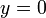$y = 0$) is a horizontal asymptote both as$x \to -\infty$ (with approach from below) and as$x \to \infty$ (with approach from above).
vertical asymptotes The$y$-axis ($x = 0$) is a vertical asymptote from both left (i.e., as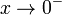$x \to 0^-$,$y \to -\infty$) and right (i.e., as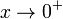$x \to 0^+$,$y \to \infty$).
first derivative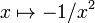$x \mapsto -1/x^2$
second derivative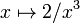$x \mapsto 2/x^3$
higher derivatives The$n^{th}$ derivative is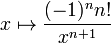$x \mapsto \frac{(-1)^nn!}{x^{n+1}}$
first antiderivative$x \mapsto \ln|x| + C$ where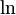$\ln$ is the natural logarithm. Note that the value$C$ is constant in$(0,\infty)$ and also constant in$(-\infty,0)$, but the constant values could differ between these two intervals.